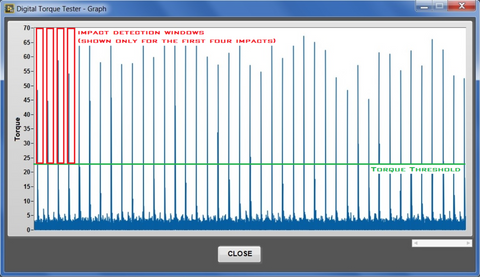White Paper – Determining Torque – Skidmore-Wilhelm

# White Paper – Determining Torque

Prepared on 08-Sep-2017

The Digital Torque Tester application operates in two modes for collecting torque data:

• Static
• Dynamic

The analysis as performed by the application will be given below for each of these two modes.

### Static Mode

The torque signal is sampled at a rate of 1,000 samples per second. Every half second, 500 samples are analyzed to provide two resultant values: (a) peak torque and (b) average torque.

Peak Torque

Every half second, the new torque values collected are compared to the peak torque found thus far during the test. If any of the new torque values are greater than the current peak torque, then this torque value becomes the new peak torque and displayed in the ‘Peak’ display box. The peak torque is set to zero when the ‘Clear Test’ button is pressed.

Average Torque

Every half second, the new torque values collected are averaged and displayed in the ‘Torque’ display box. The average torque is set to zero when the ‘Clear Test’ button is pressed.

### Dynamic Mode

The torque signal is sampled at a rate of 50,000 samples per second. The dynamic torque is processed in various steps by first determining a torque threshold and then by scanning through the data with an impact detection window.

Torque Threshold

A torque threshold is calculated as a minimum required torque for calculating the peak torque, average torque, and impacts per minute. During the first second of data collection, the maximum torque is found. For the T-1100, the torque threshold is 75% of the maximum torque found. For the T-2000 and T-3000, the torque threshold is 50% of the maximum torque found.

Impact Detection Window

The dynamic torque is continually scanned from the beginning of the test. The impact detection window finds the first torque value that is above the torque threshold. Of the next 500 samples (0.010 seconds) the maximum torque, average torque, and timestamp of the maximum torque are stored in memory. The subsequent impact detection window resumes after the next 250 samples (0.005 seconds). This process is repeated until the test ends.

As the test progresses, the peak torque is calculated from the maximum torque values stored from each impact detection window. The peak torque is simply the maximum of these maximum torque values. The maximum torque is displayed in the ‘Peak’ display box. The average torque is calculated from the average torque values stored from each impact detection window. The average torque is simply the average of these averaged torque values. The average torque is displayed in the ‘Torque’ display box. The impacts per minute is calculated from the timestamps of the maximum torque as stored from each impact detection window. The impacts per minute is displayed in the ‘Impacts/Minute’ display box.

Dynamic Torque Sample

The image below shows a sample test in dynamic torque mode. The green line indicates the Torque Threshold. The red boxes indicate the Impact Detection Windows. Please note that the impact detection windows are only shown for the first four impacts.Use this link to download a copy of the paper in PDF format.